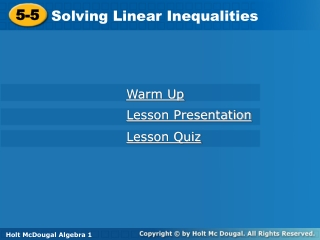DownloadDownload Presentation5-5

# 5-5

Télécharger la présentation## 5-5

- - - - - - - - - - - - - - - - - - - - - - - - - - - E N D - - - - - - - - - - - - - - - - - - - - - - - - - - -
##### Presentation Transcript

1. 5-5 Solving Linear Inequalities Warm Up Lesson Presentation Lesson Quiz Holt McDougal Algebra 1 Holt Algebra 1

2. Warm Up Graph the inequality. 1. x > –5 2. Write –6x + 2y = –4 in slope-intercept form, and graph.

3. Warm Up Graph each inequality. 1. x > –5 3. Write –6x + 2y = –4 in slope-intercept form, and graph. y = 3x – 2

4. A linear inequality is similar to a linear equation, but the equal sign is replaced with an inequality symbol. A solution of a linear inequality is any ordered pair that makes the inequality true.

5. y < 2x + 1 4 2(–2) + 1 4 –4 + 1  4 –3 < I do…. Tell whether the ordered pair is a solution of the inequality. (–2, 4); y < 2x + 1 (–2, 4) is not a solution.

6. y > x − 4 1 3 – 4 > 1 – 1 We do…. (3, 1); y > x –4 Substitute (3, 1) for (x, y).  (3, 1) is a solution.

7. 1 1 – 7 5 4 + 1 55 < 1–6 > You do… a. (4, 5); y < x + 1 b. (1, 1); y > x – 7 y < x + 1 y > x – 7   (1, 1) is a solution. (4, 5) is not a solution.

8. We do….. y = mx + b

9. We do….. y = mx + b

10. We do….. y = mx + b

11. A linear inequality describes a region of a coordinate plane called a half-plane. All points in the region are solutions of the linear inequality.

12. Solve the inequality for y (slope-intercept form). Step 1 Graph the boundary line. Use a solid line for ≤ or ≥. Use a dashed line for < or >. Step 2 Shade the half-plane above the line for y > or ≥. Shade the half-plane below the line for y < or y ≤. Check your answer. Step 3 Graphing Linear Inequalities

13. Example : Graphing Linear Inequalities in Two Variables y 2x –3 Step 1 The inequality is already solved for y. Step 2 Graph the boundary line y = 2x – 3. Use a solid line for . Step 3 The inequality is , so shade below the line.

14. Helpful Hint The point (0, 0) is a good test point to use if it does not lie on the boundary line.

15. 5x + 2y > -8 Y = mx + b

16. Check y > x – 4 0(0) – 4 0 –4  0 –4 > Example Continued 5x + 2y > –8 Substitute ( 0, 0) for (x, y).

17. Use a Grapher -Mathway grapher

18. Check It Out! 4x – y + 2 ≤ 0

19. Check It Out! 4x –3y > 12

20. Check It Out! 2x – y –4 > 0

21. Check It Out!

22. y-intercept: –5 slope: Replace = with ≤ Example : Writing an Inequality from a Graph Write an inequality to represent the graph.

23. Check It Out! Write an inequality to represent the graph. y-intercept: 0 slope: –1 y < –x.

24. Check It Out! Write an inequality to represent the graph. y-intercept: –3 slope: –2 y ≥ –2x – 3.

25. Lesson Quiz: Write an inequality to represent the graph.